# The Reuleaux Triangle and Curves of Constant Width

Why are manhole covers round? It is so they won't fall through the hole they are intended to cover. They have the same diameter regardless of where it is measured. If the hole has a slightly smaller diameter, it is not possible to orient the cover so that it will fall through. A square or rectangular cover can be turned slightly and it will easily fit through the hole.

The circle is not the only planar curve that has this constant width property. The Reuleaux Triangle is the next best-known curve of constant width. I want to describe an interactive MATLAB® program that generates generalizations of the Reuleaux Triangle.

### Contents

#### Franz Reuleaux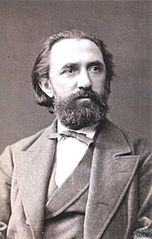Image Credit: Wikipedia

Franz Reuleaux (1829-1905) was a German mechanical engineer, a professor at the Eidgenössische Technische Hochschule Zürich, then a lecturer at the Berlin Royal Technical Academy and ultimately President of the Academy. In addition to his triangle and many other mechanical creations, he is known for the theory of kinematic pairs.

#### Reuleaux triangle

Here is the Reuleaux triangle in action in an animated gif showing one full rotation. The sides of the "triangle" are circular arcs; each is one-sixth of a circle centered at the opposite vertex.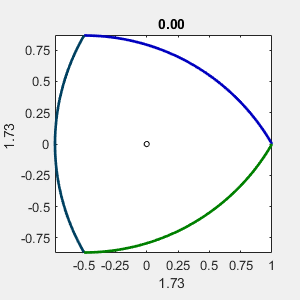The triangle is rotating, but not about the origin at its center. These plots were made with the MATLAB command

axis tight square

so the bounding box is a square with the length of a side equal to the diameter of the curve. The x and y labels show this diameter. The fact that the diameter does not change as the figure rotates is the defining property of constant width.

#### Parameters

These animations vary two parameters, n and delta. In the next two figures there are n blue dots at the vertices of a regular polygonal. There are n large arcs; each is one n-th of a circle centered at the opposite blue dot. They join the n small arcs, each is one n-th of a circle of radius delta centered at the nearby blue dot. The resulting curve is smooth, except when delta is zero.

The diameter is always the distance from one large circular arc to the opposite small circular arc, no matter how the curve is rotated.

Here n is kept constant at three and delta varies.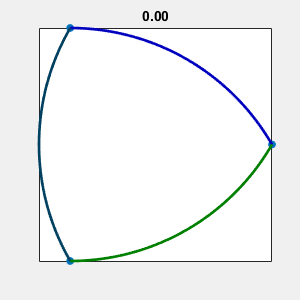Here delta is kept constant at 0.05 and n varies over odd integers.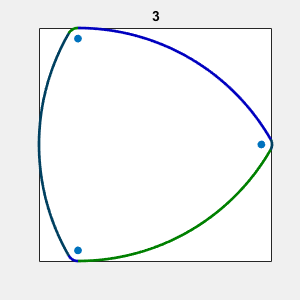#### Irregular spacing

The blue dots do not have to form a regular polygon. Here they form a 3-4-5 triangle.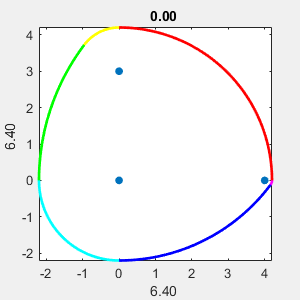#### Constant width app

I will add an interactive app, constant_width, to Cleve's Laboratory. You can choose regular or irregular spacing, vary n and delta, and rotate the resulting curves at any speed.

#### Support functions

I was intrigued by a paper by Stanley Rabinowitz. He describes how curves of constant width can be generated by so-called "support functions." An example is

$$p(\theta) = a \cos^2{(\frac{k\theta}{2})} + b$$

Parametric equations for the curve are

$$x = p(\theta) \cos{\theta} - p'(\theta) \sin{\theta}$$

$$y = p(\theta) \sin{\theta} + p'(\theta) \cos{\theta}$$

Not all choices of the parameters produce convex curves, but $a = 2$, $b = 40$, and $k = 7$ produce a satisfactory heptagonal figure. A constant step size $h = \pi/(12 k)$ yields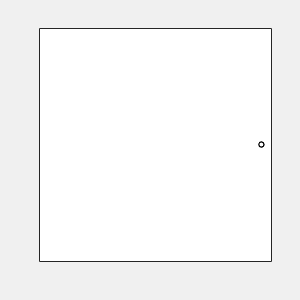Rabinowitz goes on to create a curve of constant width that is actually a polynomial of degree eight in the two variables $x$ and $y$.

#### Coins

Several countries mint coins shaped by curves of constant width. They are difficult to counterfeit and work perfectly well in vending machines. Here are a few.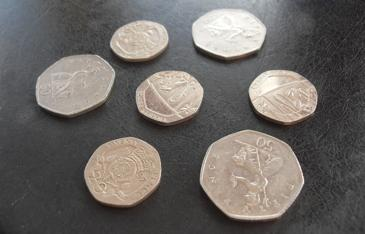Image credit: cp4space

#### Wankle rotary engine

Wankel engines, which incorporate a Reuleaux triangle, were invented in the early 1950s by Felix Wankel, a German engineer.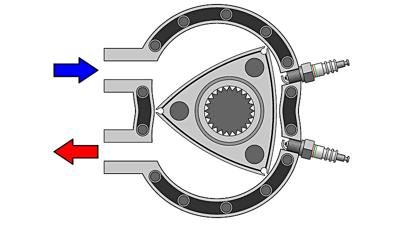Image credit: gizmodo

Published with MATLAB® R2018b

|